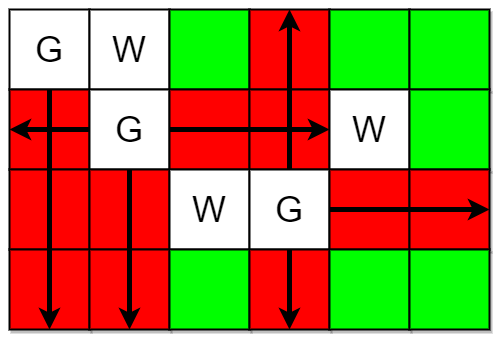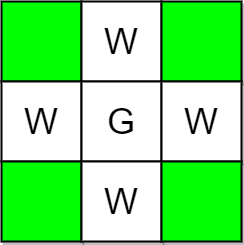# 2257. Count Unguarded Cells in the Grid

Medium
You are given two integers `m` and `n` representing a 0-indexed `m x n` grid. You are also given two 2D integer arrays `guards` and `walls` where `guards[i] = [rowi, coli]` and `walls[j] = [rowj, colj]` represent the positions of the `ith` guard and `jth` wall respectively.
A guard can see every cell in the four cardinal directions (north, east, south, or west) starting from their position unless obstructed by a wall or another guard. A cell is guarded if there is at least one guard that can see it.
Return the number of unoccupied cells that are not guarded.
Example 1:Input: m = 4, n = 6, guards = [[0,0],[1,1],[2,3]], walls = [[0,1],[2,2],[1,4]]
Output:
7
Explanation:
The guarded and unguarded cells are shown in red and green respectively in the above diagram.
There are a total of 7 unguarded cells, so we return 7.
Example 2:Input: m = 3, n = 3, guards = [[1,1]], walls = [[0,1],[1,0],[2,1],[1,2]]
Output:
4
Explanation:
The unguarded cells are shown in green in the above diagram.
There are a total of 4 unguarded cells, so we return 4.
Constraints:
• `1 <= m, n <= 105`
• `2 <= m * n <= 105`
• `1 <= guards.length, walls.length <= 5 * 104`
• `2 <= guards.length + walls.length <= m * n`
• `guards[i].length == walls[j].length == 2`
• `0 <= rowi, rowj < m`
• `0 <= coli, colj < n`
• All the positions in `guards` and `walls` are unique.

### 解題

func countUnguarded(m int, n int, guards [][]int, walls [][]int) int {
land := make([][]byte, m)
for i := range land {
land[i] = make([]byte, n)
}
for _, wall := range walls {
land[wall][wall] = 'W'
}
for _, guard := range guards {
x := guard
y := guard
land[x][y] = 'G'
for i:=x+1; i<m; i++ {
if (land[i][y]=='W') || (land[i][y]=='G') { break }
land[i][y] = 'A'
}
for i:=x-1; i>=0; i-- {
if (land[i][y]=='W') || (land[i][y]=='G') { break }
land[i][y] = 'A'
}
for j:=y+1; j<n; j++ {
if (land[x][j]=='W') || (land[x][j]=='G') { break }
land[x][j] = 'A'
}
for j:=y-1; j>=0; j-- {
if (land[x][j]=='W') || (land[x][j]=='G') { break }
land[x][j] = 'A'
}
}
ans := 0
for i:=0; i<m; i++ {
for j:=0; j<n; j++ {
if land[i][j]!='W' && land[i][j]!='G' && land[i][j]!='A' {
ans++
}
}
}
return ans
}